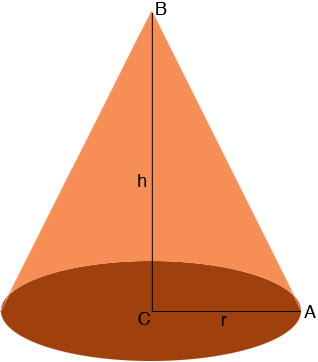SEARCH HOMEMath Central Quandaries & QueriesQuestion from Linda, a parent: How do you calculate the volume of a cone that is 25cm high and has an angle of 20 degrees?Hi Linda,

The volume of a cone of base radius $r$ cm and height $h$ cm is

$\frac13 \pi\; r^2 h.$

You know the height so you need to find the radius of the base.In my diagram the triangle ABC is a right triangle and the measure of angle CAB is 20 degrees. What trig function connects the lengths of the sides BC and CA with the measure of the angle CAB?

PennyMath Central is supported by the University of Regina and the Imperial Oil Foundation.